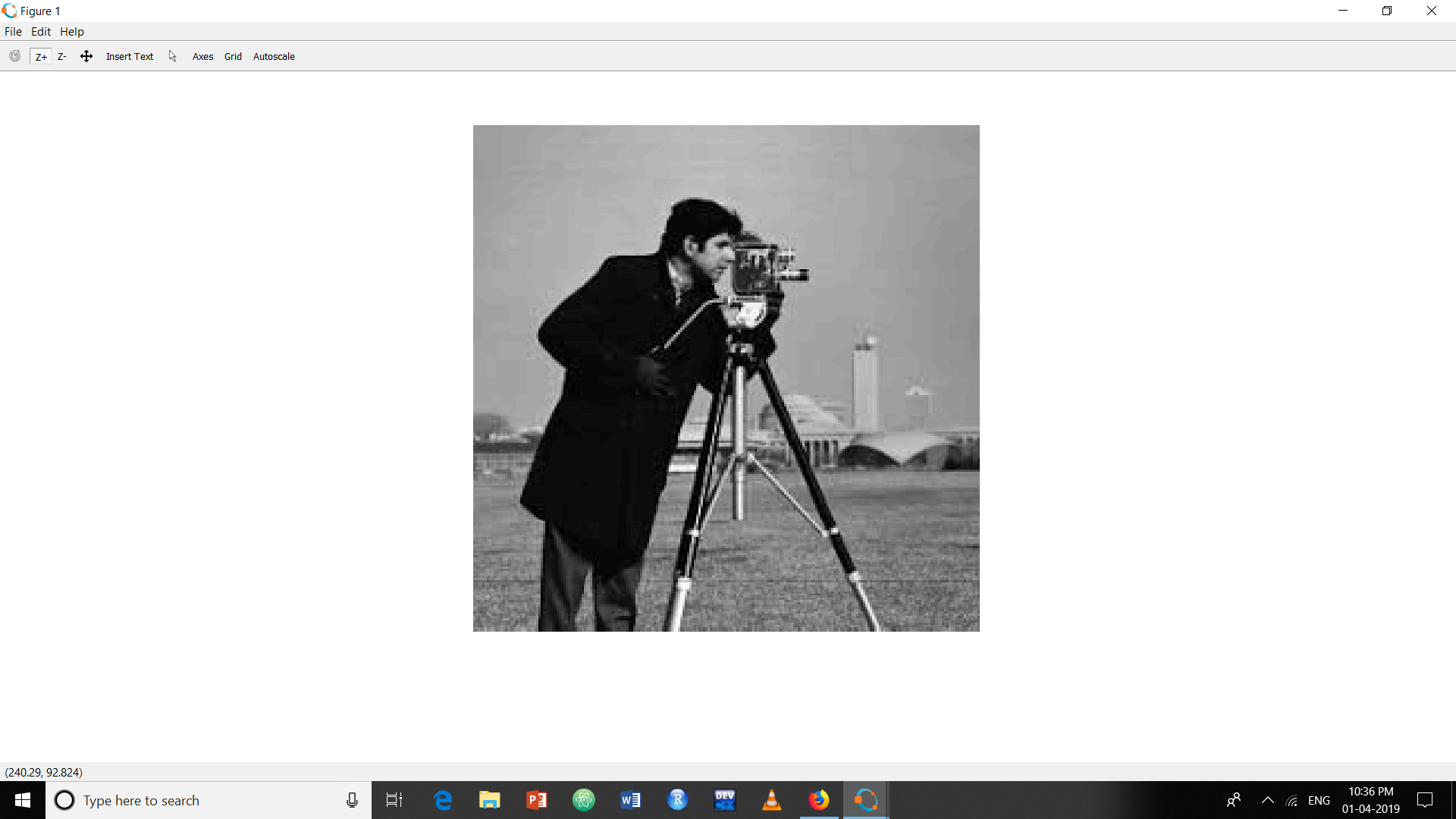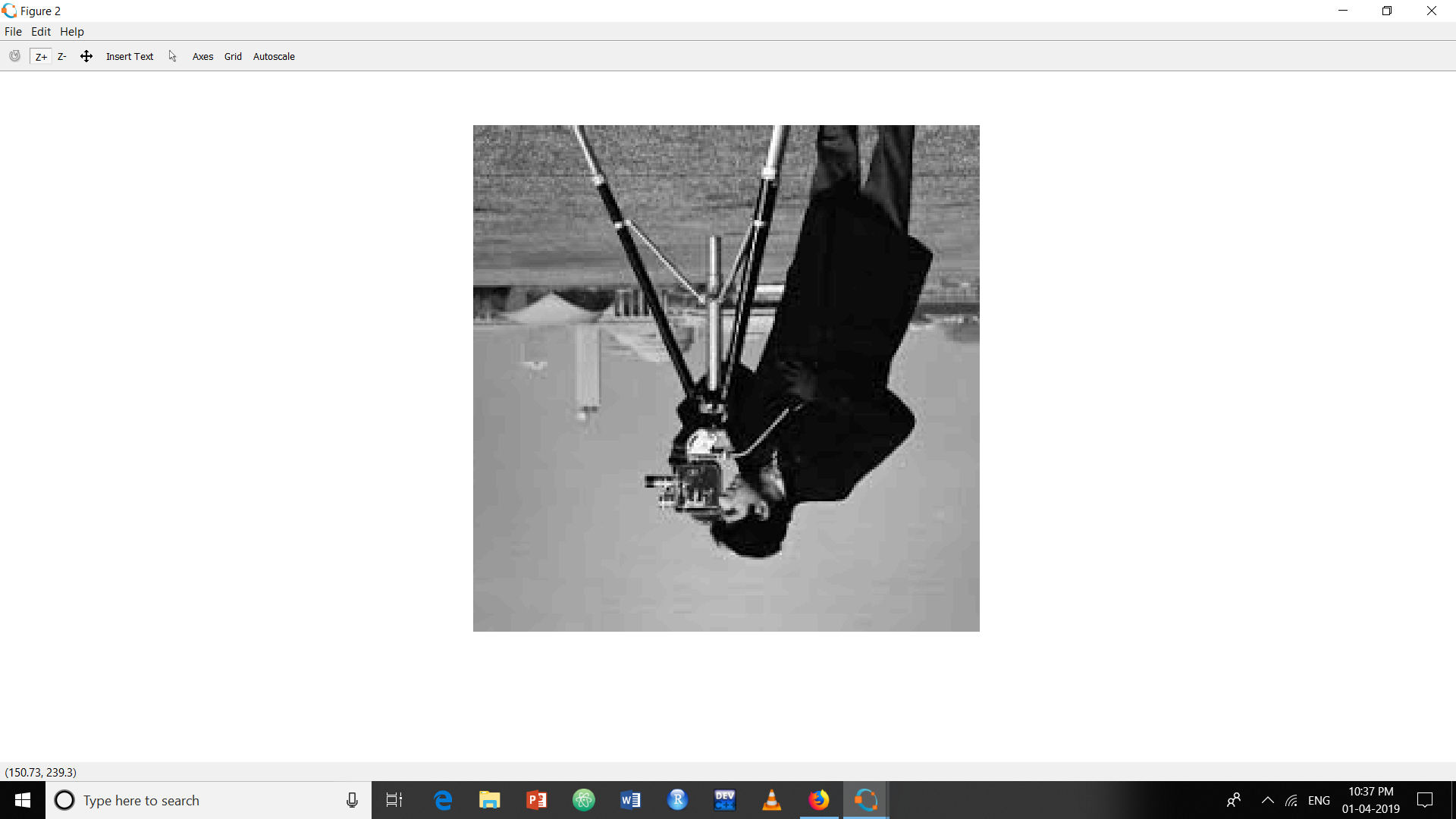Skip to content
Related Articles
Matlab program to rotate an image 180 degrees clockwise without using function
• Last Updated : 07 Aug, 2020

An image is defined as two-dimensional function,` f(x, y)`, where x and y are spatial (plane) coordinates, and the amplitude of f at any pair of `coordinates(x, y)` is called the Intensity or the Gray level of the image at that point. When x, y and the intensity values of f are all finite, discrete quantities, the image is digital image.

Digital image is composed of a finite number of elements, each of which has a particular location and value. These elements are called picture elements, image elements and pixels. Pixel is the smallest element of an image. Each pixel corresponds to any one value. For an 8-bit gray scale image, the value of the pixel is between 0-255.

## Image Rotation –

The rows are rotated from end to -1 keeping the step size 1. To rotate the image clockwise, the columns are rotated from end to -1 keeping the step size 1.

Approach:

• Read the image using imread function.
• Show the image using imshow function.
• Rotate the image.
• Display the image using imshow.

Below is the implementation:

 `% Read the Image``a = imread(``"cameraman.png"``);`` ` `% Display the image``imshow(a);`` ` `% Rotate the image clockwise``i = a(``end``:-1 : 1, ``end``:-1 : 1);`` ` `% Display the rotated image``figure, imshow(i);`

Output:

Original Image –Rotated Image –My Personal Notes arrow_drop_up